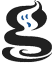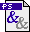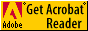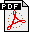# McMaster UniversityLogic and Discrete Mathematics in Software Engineering

## Lecture Notes

### 12 September:

• Notation for quantification and set comprehension taken from the Formal Specification Notation Z (see also Wikipedia: Z notation)
• Relations as subsets of cartesian products; composition of binary relations
• Orders:
• Point-wise and point-free definitions of reflexivity, transitivity, and antisymmetry
• Hasse diagrams
• Duality
• Upper bounds, maxima, greatest elements, least upper bounds, and their duals
• Order-theoretic definition
• Examples, including (N, ≤) and (N, |)
• Algebraic definition
• Definition of the ordering in the algebraic setting
• Example: Concept Lattices

### 17 September:

• Proving idempotence and order properties in algebraic lattices
• Functions and related concepts (univalent, total, mapping, injective, surjective, bijective)
• point-free relation-algebraic definitions
• arrow symbols from the Z notation
• Monotonicity
• monotonic mappings as order homomorphisms
• the inverse of a bijective order homomorphism is not necessarily monotonic
• Definition of closure operators (see also Wikipedia on closure and closure operator)
• Recognition of extent and intent closure operators in the Concept Lattice paper

### 19 September:

• Proving monotoniciy of the composition of monotonic mappings
• Atoms in partial orders with least elements
• Atomic lattices, join-irreducible elements
• Complete lattices
• Knaster-Tarski fixpoint theorem (see also Wikipedia — that page also contains a link to more on-line material on lattices)
• Relative complement (correction): In a lattice with least element ⊥, an element A is called relative complement of the element B with respect to the element T iff A is the least element such that  AB = ⊥  and  ABT.
• Complement (not in class): In a lattice with least element ⊥ and greatest element Τ, an element A is called complement of the element B iff  AB = ⊥  and  AB ≥ Τ.

### 24 September:

• Lattices:
• Definition of sublattice
• Modular and distributive lattices
• sublattices documenting non-modularity and non-distributivity
• Complements in lattices
• non-uniqueness of complements in simple non-modular and non-distributive lattices
• absence of complement for the middle elements of linear orders
• Formal Languages Crash Course:

### 26 September:

• Relations:
• Definition of reflexive transitive closure
• Care with well-definedness
• Pre-orders, induced equivalence relations, induced order on the quotient
• Formal Languages: Discussion of string grammars versus tree grammars (for example DTDs)
• Logic: General discussion of syntax versus semantics, syntactic derivability versus semantic entailment, soundness and completeness.

### 1 October:(-Viewer)(-Viewer)